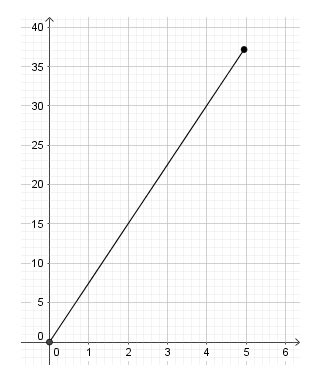# Proportional Relationships

## Objective

Make connections between the four representations of proportional relationships (Part 2).

## Common Core Standards

### Core Standards

?

• 7.RP.A.2 — Recognize and represent proportional relationships between quantities.

• 7.RP.A.2.A — Decide whether two quantities are in a proportional relationship, e.g., by testing for equivalent ratios in a table or graphing on a coordinate plane and observing whether the graph is a straight line through the origin.

• 7.RP.A.2.B — Identify the constant of proportionality (unit rate) in tables, graphs, equations, diagrams, and verbal descriptions of proportional relationships.

• 7.RP.A.2.C — Represent proportional relationships by equations. For example, if total cost t is proportional to the number n of items purchased at a constant price p, the relationship between the total cost and the number of items can be expressed as t = pn.

• 7.RP.A.2.D — Explain what a point (x, y) on the graph of a proportional relationship means in terms of the situation, with special attention to the points (0, 0) and (1, r) where r is the unit rate.

?

• 6.RP.A.3

## Criteria for Success

?

1. Represent and analyze proportional relationships in graphs, equations, and tables.
2. Given one representation of a proportional relationship, create other representations.

## Tips for Teachers

?

The Problem Set Guidance describes a possible activity to help students make connections between the representations. This is a good opportunity for students to showcase their collective work and look at the work of others, via posters, a gallery walk, or in small groups.

#### Fishtank Plus

• Problem Set
• Student Handout Editor
• Vocabulary Package

## Anchor Problems

?

### Problem 1

A proportional relationship is shown in the graph below.

1. Describe a situation that could be represented with this graph.
2. Write an equation for the relationship. Explain what each part of the equation represents.### Problem 2

Jonathan and his brother Jeffrey went on a long run to prepare for an upcoming road race. Jonathan ran 18 miles in 4 hours. It took Jeffrey 6 hours to run the same route as his brother. Both brothers ran at a constant speed.

1. Draw a graph to represent the relationship between distance and time for the two brothers. Label each line with the brother’s name.
2. Explain what the graph tells you about the speed of each brother.
3. The road race is a marathon and is 26.2 miles long. If Jonathan and Jeffrey each run at the same constant speed as they did in their preparation run, then how long will it take each brother to finish the road race?

## Problem Set

?The following resources include problems and activities aligned to the objective of the lesson that can be used to create your own problem set.

• Have students create one representation of a proportional relationship (a table, written description, graph, or equation). Then have students swap their work with other students who add a new representation to the one already provided. This can be repeated so that, after 4 students have worked on each situation, all four representations of the proportional relationship are shown.

Patrice makes a spicy salsa by adding red pepper flakes to a chunky tomato mix in proportional amounts. For example, she mixes $\frac{1}{2}$ teaspoon of red pepper flakes to 2 cups of tomato mix.